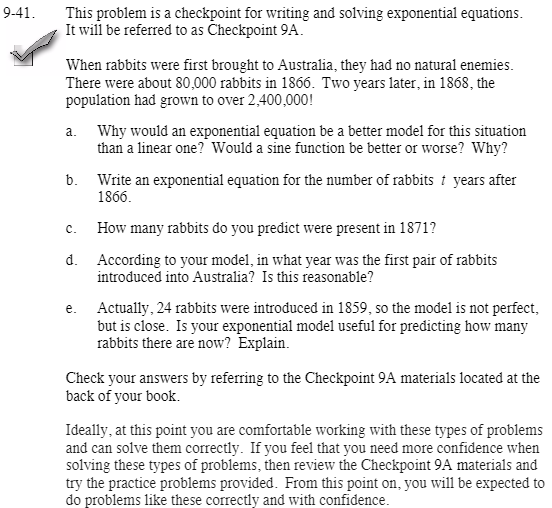Home > CCA2 > Chapter 9 > Lesson 9.1.3 > Problem9-41

9-41.
1.This problem is a checkpoint for writing and solving exponential equations. It will be referred to as Checkpoint 9A.

2. When rabbits were first brought to Australia, they had no natural enemies. There were about 80,000 rabbits in 1866. Two years later, in 1868, the population had grown to over 2,400,000! Homework Help ✎

1. Why would an exponential equation be a better model for this situation than a linear one? Would a sine function be better or worse? Why?

2. Write an exponential equation for the number of rabbits t years after 1866.

3. How many rabbits do you predict were present in 1871?

4. According to your model, in what year was the first pair of rabbits introduced into Australia? Is this reasonable?

5. Actually, 24 rabbits were introduced in 1859, so the model is not perfect, but is close. Is your exponential model useful for predicting how many rabbits there are now? Explain.

3. Check your answers by referring to the Checkpoint 9A materials.

4. Ideally, at this point you are comfortable working with these types of problems and can solve them correctly. If you feel that you need more confidence when solving these types of problems, then review the Checkpoint 9A materials and try the practice problems provided. From this point on, you will be expected to do problems like these correctly and with confidence.Answers and extra practice for the Checkpoint problems are located in the back of your printed textbook or in the Reference Tab of your eBook. If you have an eBook for CCA2, login and then click the following link: Checkpoint 9A: Writing and Solving Exponential Equations